# Build a Map with Lidar Odometry and Mapping (LOAM) Using Unreal Engine Simulation

This example shows how to build a map with the lidar odometry and mapping (LOAM)  algorithm by using synthetic lidar data from the Unreal Engine® simulation environment. In this example, you learn how to:

• Record and visualize synthetic lidar sensor data from a 3D simulation environment using the Unreal Engine.

• Use the LOAM algorithm to register the recorded point clouds and build a map.

### Set Up Scenario in Simulation Environment

Load the prebuilt Large Parking Lot (Automated Driving Toolbox) scene and a preselected reference trajectory. For information on how to generate a reference trajectory interactively by selecting a sequence of waypoints, see the Select Waypoints for Unreal Engine Simulation (Automated Driving Toolbox) example.

```% Load reference path data = load("parkingLotReferenceData.mat"); % Set reference trajectory of the ego vehicle refPosesX = data.refPosesX; refPosesY = data.refPosesY; refPosesT = data.refPosesT; % Set poses of the parked vehicles parkedPoses = data.parkedPoses; % Display the reference trajectory and the parked vehicle locations sceneName = "LargeParkingLot"; hScene = figure(Name="Large Parking Lot",NumberTitle="off"); helperShowSceneImage(sceneName); hold on plot(refPosesX(:,2),refPosesY(:,2),LineWidth=2,DisplayName="Reference Path"); scatter(parkedPoses(:,1),parkedPoses(:,2),[],"filled",DisplayName="Parked Vehicles"); xlim([-60 40]) ylim([10 60]) hScene.Position = [100 100 1000 500]; % Resize figure title("Large Parking Lot") legend```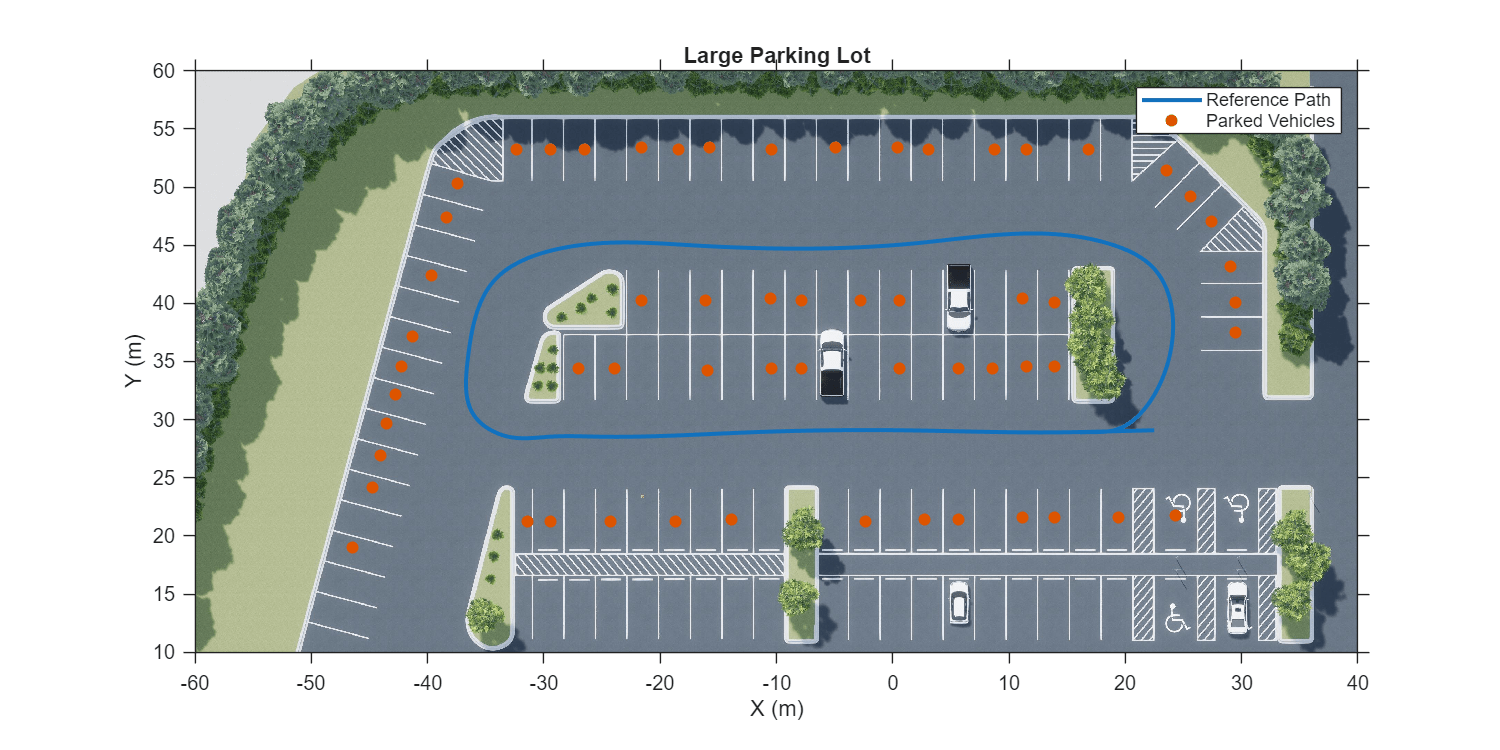Open the Simulink® model, and add additional vehicles to the scene using the `helperAddParkedVehicle` function.

```modelName = 'GenerateLidarDataOfParkingLot'; open_system(modelName) snapnow helperAddParkedVehicles(modelName,parkedPoses)```

### Record and Visualize Data

Use the Simulation 3D Vehicle with Ground Following (Automated Driving Toolbox) block to simulate a vehicle moving along the specified reference trajectory. Use the Simulation 3D Lidar (Automated Driving Toolbox) block to mount a lidar on the center of the roof of the vehicle, and record the sensor data.

```close(hScene) if ~ispc error("Unreal Engine Simulation is supported only on Microsoft" + char(174) + " Windows" + char(174) + "."); end % Run simulation simOut = sim(modelName); close_system(modelName,0)```

Use the `helperGetPointClouds` function and the `helperGetLidarGroundTruth` function to extract the lidar data and the ground truth poses.

```ptCloudArr = helperGetPointClouds(simOut); groundTruthPosesLidar = helperGetLidarGroundTruth(simOut);```

### Detect Edge Points and Surface Points

The LOAM algorithm uses edge points and surface points for registration and mapping. The `detectLOAMFeatures` function outputs a `LOAMPoints` object, which stores the selected edge points and surface points. It includes the label of each point, which can be sharp-edge, less-sharp-edge, planar-surface, or less-planar-surface. Use the `pcregisterloam` function to register two organized point clouds.

```ptCloud = ptCloudArr(1); nextPtCloud = ptCloudArr(2); gridStep = 0.5; tform = pcregisterloam(ptCloud,nextPtCloud,gridStep); disp(tform)```
``` rigidtform3d with properties: Dimensionality: 3 Translation: [-0.2765 2.5534 -0.6325] R: [3×3 single] A: [4×4 single] ```

Alternatively, for more control over the trade-off between accuracy and speed, you can first detect the LOAM feature points, and then perform LOAM registration using `pcregisterloam`. These steps are recommended before LOAM registration:

1. Detect LOAM feature points using the `detectLOAMFeatures` function.

2. Downsample the less planar surface points using the `downsampleLessPlanar` object function. This step helps speed up registration using the `pcregisterloam` function.

Because the detection algorithm relies on the neighbors of each point to classify edge points and surface points, as well as to identify unreliable points on the boundaries of occluded regions, preprocessing steps like downsampling, denoising and ground removal are not recommended before feature point detection. To remove noise from data farther from the sensor, and to speed up registration, filter the point cloud by range. The `findPointsInCylinder` object function selects a cylindrical neighborhood around the sensor, given a specified cylinder radius, and excludes data that is too close to the sensor and might include part of the vehicle.

```egoRadius = 3.5; cylinderRadius = 50; selectedIdx = findPointsInCylinder(ptCloud,[egoRadius cylinderRadius]); ptCloud = select(ptCloud,selectedIdx,OutputSize="full"); selectedIdx = findPointsInCylinder(nextPtCloud,[egoRadius cylinderRadius]); nextPtCloud = select(nextPtCloud,selectedIdx,OutputSize="full"); figure hold on title("Cylindrical Neighborhood") pcshow(ptCloud) view(2)```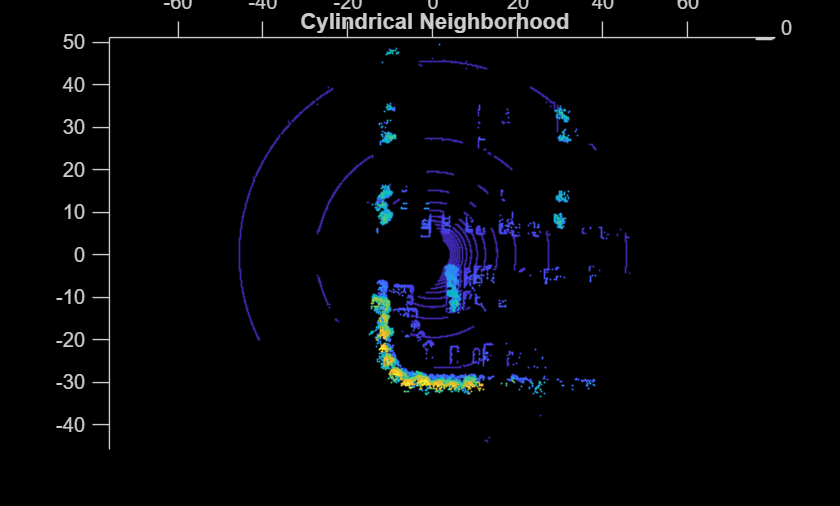Next, detect LOAM feature points using the `detectLOAMFeatures` function. Tuning this function requires empirical analysis. The `detectLOAMFeatures` name-value arguments provide a trade-off between registration accuracy and speed. To improve the accuracy of the registration, you must minimize the root mean squared error of the Euclidean distance between the aligned points. Track and minimize the root mean squared error output `rmse` of the `pcregisterloam` function as you increase the value of the `NumRegionsPerLaser`, `MaxSharpEdgePoints`, `MaxLessSharpEdgePoints`, and `MaxPlanarSurfacePoints` arguments of `detectLOAMFeatures`.

```maxPlanarSurfacePoints = 8; points = detectLOAMFeatures(ptCloud,MaxPlanarSurfacePoints=maxPlanarSurfacePoints); nextPoints = detectLOAMFeatures(nextPtCloud,MaxPlanarSurfacePoints=maxPlanarSurfacePoints); figure hold on title("LOAM Points") show(points,MarkerSize=12)```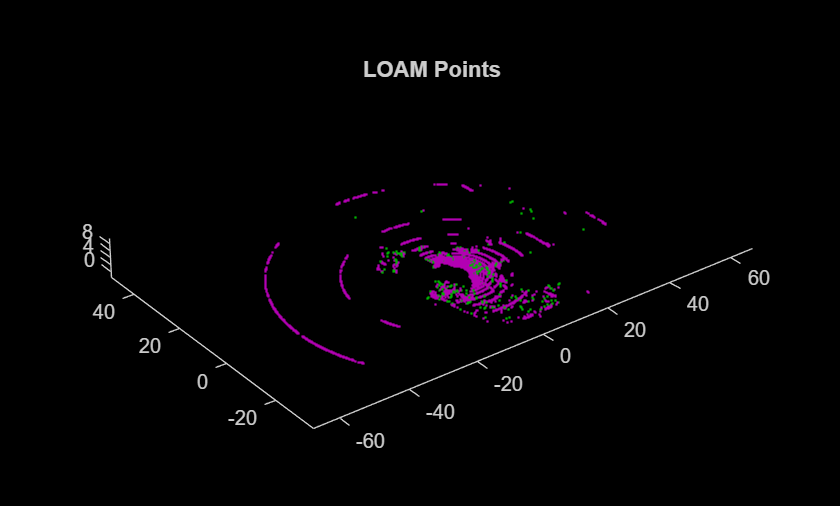```[~,rmseValue] = pcregisterloam(points,nextPoints); disp(['RMSE: ' num2str(rmseValue)])```
```RMSE: 0.59803 ```

`detectLOAMFeatures` first identifies sharp edge points, less sharp edge points, and planar surface points. All remaining points that are not considered unreliable points, and have a curvature value below the threshold are classified as less planar surface points. Downsampling the less planar surface points can speed up registration when using `pcregisterloam`.

```points = downsampleLessPlanar(points,gridStep); figure hold on title('LOAM Points After Downsampling the Less Planar Surface Points') show(points,'MarkerSize',12)```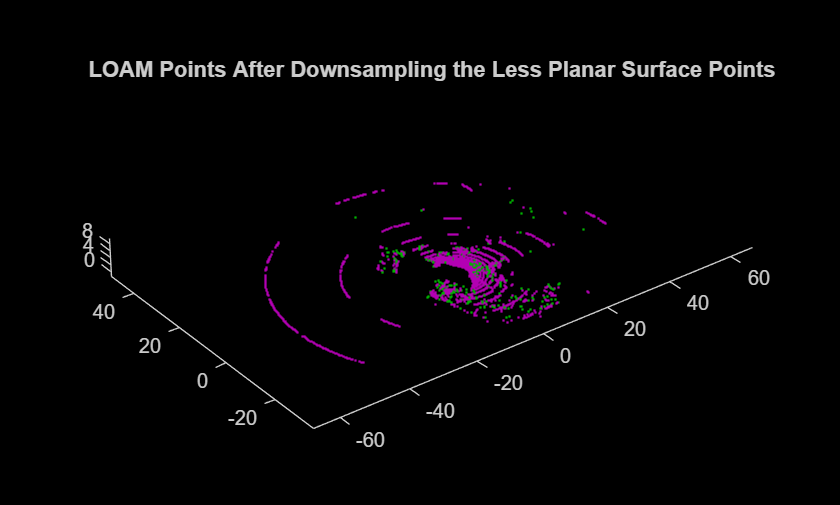### Build Map Using Lidar Odometry

The LOAM algorithm consists of two main components that are integrated to compute an accurate transformation: Lidar Odometry and Lidar Mapping. Use the `pcregisterloam` function with the one-to-one matching method to get the estimated transformation using the Lidar Odometry algorithm. The one-to-one matching method matches each point to its nearest neighbor, matching edge points to edge points and surface points to surface points. Use these matches to compute an estimate of the transformation. Use `pcregisterloam` with the one-to-one matching method to incrementally build a map of the parking lot. Use a `pcviewset` object to manage the data.

Initialize the poses and the point cloud view set.

```absPose = groundTruthPosesLidar(1); relPose = rigidtform3d; vSetOdometry = pcviewset;```

Add the first view to the view set.

```viewId = 1; vSetOdometry = addView(vSetOdometry,viewId,absPose);```

Register the point clouds incrementally and visualize the vehicle position in the parking lot scene.

```% Display the parking lot scene with the reference trajectory hScene = figure(Name="Large Parking Lot",NumberTitle="off"); helperShowSceneImage(sceneName); hold on plot(refPosesX(:,2),refPosesY(:,2),LineWidth=2) xlim([-60 40]) ylim([10 60]) hScene.Position = [100 100 1000 500]; numSkip = 5; for k = (numSkip+1):numSkip:numel(ptCloudArr) prevPoints = points; viewId = viewId + 1; ptCloud = ptCloudArr(k); % Select a cylindrical neighborhood of interest. selectedIdx = findPointsInCylinder(ptCloud,[egoRadius cylinderRadius]); ptCloud = select(ptCloud,selectedIdx,OutputSize="full"); % Detect LOAM points and downsample the less planar surface points points = detectLOAMFeatures(ptCloud,MaxPlanarSurfacePoints=maxPlanarSurfacePoints); points = downsampleLessPlanar(points,gridStep); % Register the points using the previous relative pose as an initial % transformation relPose = pcregisterloam(points,prevPoints,InitialTransform=relPose); % Update the absolute pose and store it in the view set absPose = rigidtform3d(absPose.A * relPose.A); vSetOdometry = addView(vSetOdometry,viewId,absPose); % Visualize the absolute pose in the parking lot scene plot(absPose.Translation(1),absPose.Translation(2),Color="r",Marker=".",MarkerSize=8); xlim([-60 40]) ylim([10 60]) title("Build a Map Using Lidar Odometry") legend(["Ground Truth","Estimated Position"]) pause(0.001) view(2) end```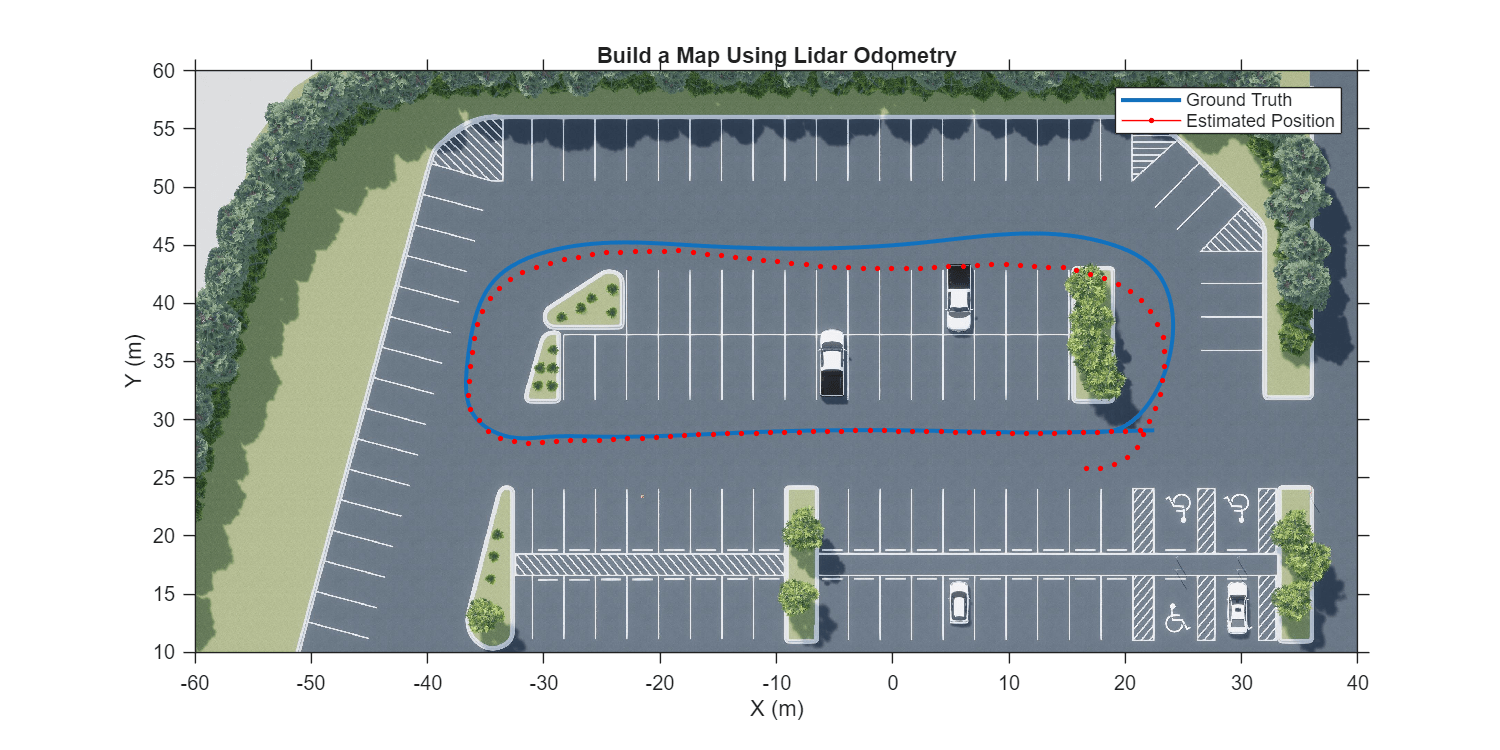### Improve the Accuracy of the Map with Lidar Mapping

Lidar Mapping refines the pose estimate from Lidar odometry by doing registration between points in a laser scan and points in a local map that includes multiple laser scans. It matches each point to multiple nearest neighbors in the local map, and then it uses these matches to refine the transformation estimate from Lidar odometry. Use the `pcmaploam` object to manage the points in the map. Refine the pose estimates from Lidar odometry using `findPose`, and add points to the map using `addPoints``.`

Initialize the poses.

```absPose = groundTruthPosesLidar(1); relPose = rigidtform3d; vSetMapping = pcviewset;```

Detect LOAM points in the first point cloud.

```ptCloud = ptCloudArr(1); selectedIdx = findPointsInCylinder(ptCloud,[egoRadius cylinderRadius]); ptCloud = select(ptCloud,selectedIdx,OutputSize="full"); points = detectLOAMFeatures(ptCloud,'MaxPlanarSurfacePoints',maxPlanarSurfacePoints); points = downsampleLessPlanar(points,gridStep);```

Add the first view to the view set.

```viewId = 1; vSetMapping = addView(vSetMapping,viewId,absPose);```

Create a map using the `pcmaploam` class, and add points to the map using the `addPoints` object function of `pcmaploam`.

```voxelSize = 0.1; loamMap = pcmaploam(voxelSize); addPoints(loamMap,points,absPose);```

Use `pcregisterloam` with the one-to-one matching method to get an estimated pose using Lidar Odometry. Then, use the `findPose` object function of `pcmaploam` to find the absolute pose that aligns the points to the points in the map.

```% Display the parking lot scene with the reference trajectory hScene = figure(Name="Large Parking Lot",NumberTitle="off"); helperShowSceneImage(sceneName); hold on plot(refPosesX(:,2),refPosesY(:,2),LineWidth=2) xlim([-60 40]) ylim([10 60]) hScene.Position = [100 100 1000 500]; for k = (numSkip+1):numSkip:numel(ptCloudArr) prevPtCloud = ptCloud; prevPoints = points; viewId = viewId + 1; ptCloud = ptCloudArr(k); % Select a cylindrical neighborhood of interest. selectedIdx = findPointsInCylinder(ptCloud,[egoRadius cylinderRadius]); ptCloud = select(ptCloud,selectedIdx,OutputSize="full"); % Detect LOAM points and downsample the less planar surface points points = detectLOAMFeatures(ptCloud,MaxPlanarSurfacePoints=maxPlanarSurfacePoints); points = downsampleLessPlanar(points,gridStep); % Register the points using the previous relative pose as an initial % transformation relPose = pcregisterloam(points,prevPoints,MatchingMethod="one-to-one",InitialTransform=relPose); % Find the refined absolute pose that aligns the points to the map absPose = findPose(loamMap,points,relPose); % Store the refined absolute pose in the view set vSetMapping = addView(vSetMapping,viewId,absPose); % Add the new points to the map addPoints(loamMap,points,absPose); % Visualize the absolute pose in the parking lot scene plot(absPose.Translation(1),absPose.Translation(2),Color="r",Marker=".",MarkerSize=8) xlim([-60 40]) ylim([10 60]) title("Build a Map Using Lidar Mapping") legend(["Ground Truth","Estimated Position"]) pause(0.001) view(2) end```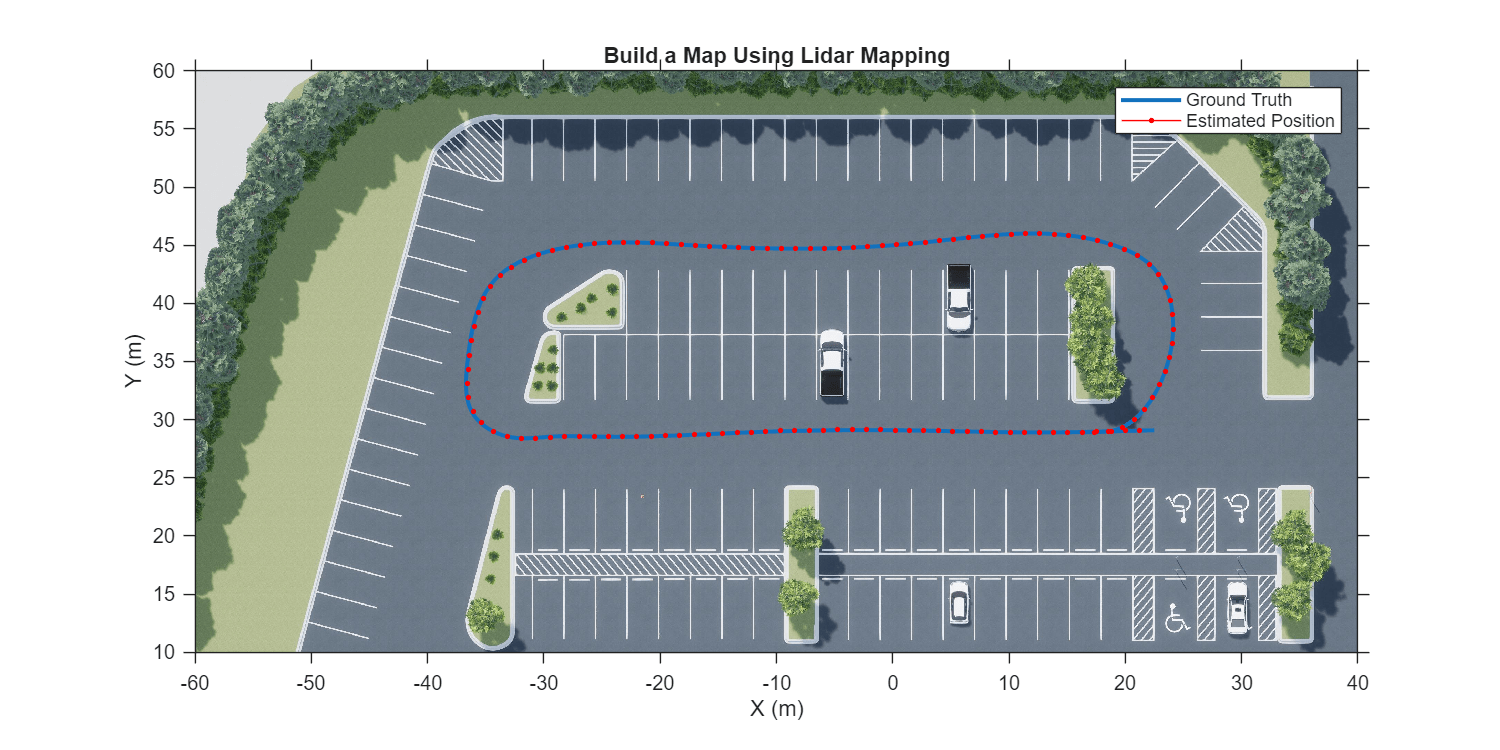### Compare Results

Visualize the estimated trajectories and compare them to the ground truth. Notice that combining Lidar Odometry and Lidar Mapping results in a more accurate map.

```% Get the ground truth trajectory groundTruthTrajectory = vertcat(groundTruthPosesLidar.Translation); % Get the estimated trajectories odometryPositions = vertcat(vSetOdometry.Views.AbsolutePose.Translation); mappingPositions = vertcat(vSetMapping.Views.AbsolutePose.Translation); % Plot the trajectories figure plot3(groundTruthTrajectory(:,1),groundTruthTrajectory(:,2),groundTruthTrajectory(:,3),LineWidth=2,DisplayName="Ground Truth") hold on plot3(odometryPositions(:,1),odometryPositions(:,2),odometryPositions(:,3),LineWidth=2,DisplayName="Odometry") plot3(mappingPositions(:,1),mappingPositions(:,2),mappingPositions(:,3),LineWidth=2,DisplayName="Odometry and Mapping") view(2) legend title("Compare Trajectories")```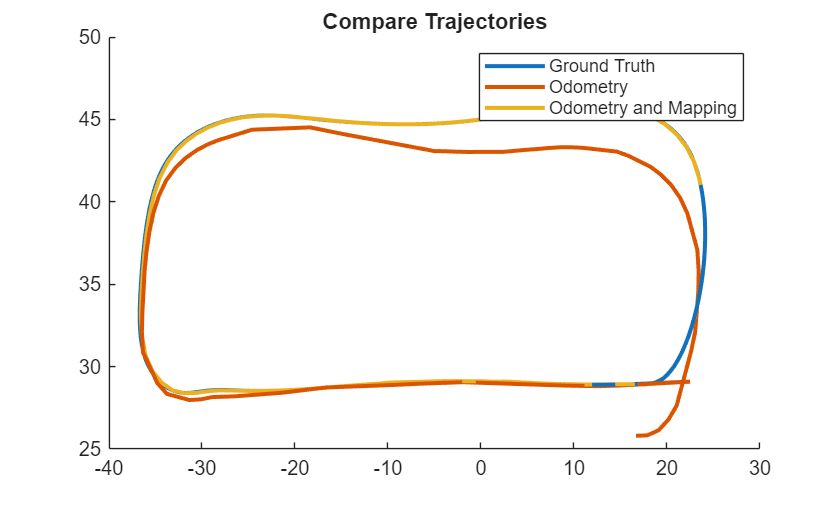To numerically compare the estimated trajectories with the ground truth, compute the root-mean-square error (RMSE) between the ground truth trajectory and the estimated trajectory.

```% Select the poses to compare selectedGroundTruth = groundTruthTrajectory(1:numSkip:end,:); % Compute the RMSE rmseOdometry = rmse(selectedGroundTruth,odometryPositions,"all"); rmseWithMapping = rmse(selectedGroundTruth,mappingPositions,"all"); disp(['RMSE of the trajectory estimated with Odometry: ' num2str(rmseOdometry)])```
```RMSE of the trajectory estimated with Odometry: 1.1396 ```
`disp(['RMSE of the trajectory estimated with Odometry and Mapping: ' num2str(rmseWithMapping)])`
```RMSE of the trajectory estimated with Odometry and Mapping: 0.2185 ```

### References

 Zhang, Ji, and Sanjiv Singh. “LOAM: Lidar Odometry and Mapping in Real-Time.” In Robotics: Science and Systems X. Robotics: Science and Systems Foundation, 2014. https://doi.org/10.15607/RSS.2014.X.007.

### Supporting Functions

`helperGetPointClouds` extracts an array of `pointCloud` objects that contain lidar sensor data.

```function ptCloudArr = helperGetPointClouds(simOut) % Extract signal ptCloudData = simOut.ptCloudData.signals.values; % Create a pointCloud array ptCloudArr = pointCloud(ptCloudData(:,:,:,2)); % Ignore first frame for n = 1:size(ptCloudData,4) ptCloudArr(end+1) = pointCloud(ptCloudData(:,:,:,n)); %#ok<AGROW> end end```

`helperGetLidarGroundTruth` extracts an array of `rigidtform3d` objects that contain the ground truth location and orientation.

```function gTruth = helperGetLidarGroundTruth(simOut) numFrames = size(simOut.lidarLocation.time,1); gTruth = repmat(rigidtform3d,numFrames-1,1); for i = 1:numFrames gTruth(i).Translation = squeeze(simOut.lidarLocation.signals.values(:,:,i)); % Ignore the roll and the pitch rotations since the ground is flat yaw = double(simOut.lidarOrientation.signals.values(:,3,i)); gTruth(i).R = [cos(yaw) -sin(yaw) 0; sin(yaw) cos(yaw) 0; 0 0 1]; end end```